• 主要介绍了用Python实现随机森林算法，小编觉得挺不错的，现在分享给大家，也给大家做个参考。一起跟随小编过来看看吧
import pandas as pd
import numpy as np
from sklearn.ensemble import RandomForestClassifier
import matplotlib.pyplot as plt

train=pd.read_excel('E:/project_6.18/train.xlsx')

特征处理 非尖货–1 尖货–0 价格力等级 低–0 中–1 高–2
#处理是否尖货
train_f['是否尖货']=(train_f['是否尖货']=='非尖货').astype('int')
#处理价格力等
labels=train_f['价格力等级'].unique().tolist()#显示唯一的值
train_f['价格力等级']=train_f['价格力等级'].apply(lambda x : labels.index(x))
train_f.drop(['商品id'],axis=1,inplace=True)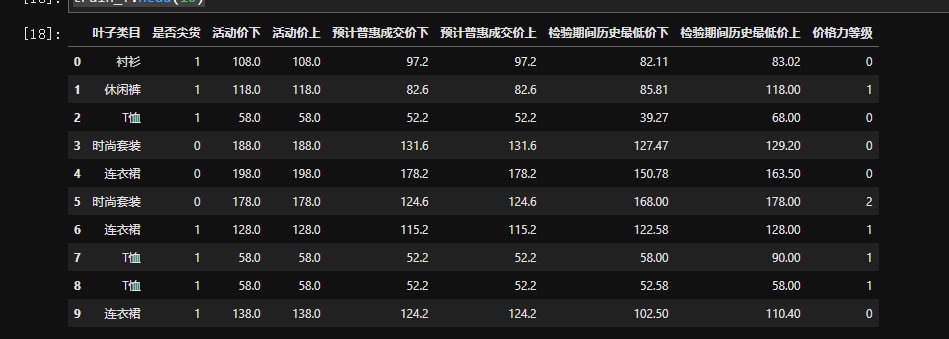#对叶子类目做二值化分列处理
train_f = train_f.join(pd.get_dummies(train_f.叶子类目, prefix= '叶子类目'))
#建模特征
train_f_y=train_f['价格力等级']
train_f_x=train_f.drop(['叶子类目','价格力等级'],axis=1)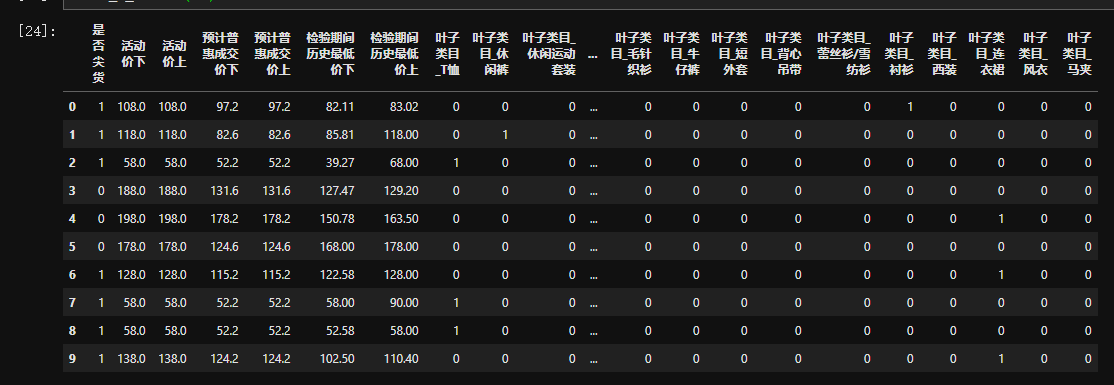测试集
#处理是否尖货
test['是否尖货']=(test['是否尖货']=='非尖货').astype('int')
#处理价格力等
test['价格力等级']=test['价格力等级'].apply(lambda x : labels.index(x))

test.drop(['商品id'],axis=1,inplace=True)
#对叶子类目做二值化分列处理
test= test.join(pd.get_dummies(test.叶子类目, prefix= '叶子类目'))
#建模特征
test_x=test.drop(['叶子类目','价格力等级'],axis=1)


建模（训练集得分）
forest=RandomForestClassifier(n_estimators=100,random_state=0,n_jobs=-1)
forest.fit(train_f_x,train_f_y)
test_y=forest.predict(test_x)
train_score=forest.score(train_f_x,train_f_y)
print(train_score)特征重要性
feature_imp_sorted_rf = pd.DataFrame({'feature': list(train_f_x), 'importance': forest.feature_importances_}).sort_values('importance', ascending=False)
feature_imp_sorted_rf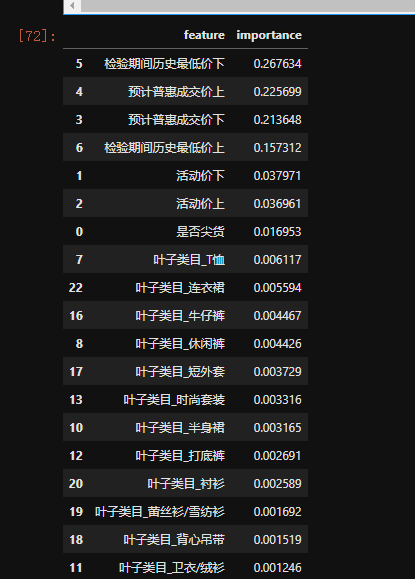测试集得分 方1
test_score=forest.score(test_x,answer_y)
test_score

方2
from sklearn.metrics import mean_squared_error
1-score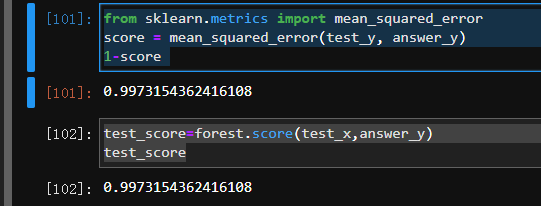查看预测错误的（有6个）
answer_y=pd.DataFrame(answer_y)
pred=pd.DataFrame(test_y.tolist())
sco['cha']=sco['价格力等级']-sco
sco[sco['cha']!=0]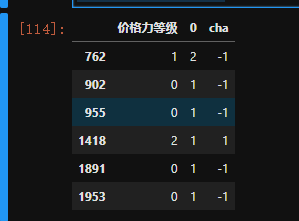展开全文• 随机森林是数据挖掘中非常常用的分类预测算法，以分类或回归的决策树为基分类器。算法的一些基本要点： *对大小为m的数据集进行样本量同样为m的有放回抽样； *对K个特征进行随机抽样，形成特征的子集，样本量的确定...
• 本文件包括随机森林的代码实现和相应的数据集，以及详尽的...因为只是用来学习随机森林算法，所以在调参方法没下多少功夫，正确率可能不太高,当然数据集比较小也是一个原因。感兴趣的童鞋可以自己调整参数提高正确率。
• 随机森林算法 决策树算法可以很容易的将模型进行可视化，同时它是将每个样本特征进行单独处理，故而不需要的对数据进行转换。但是决策树会很容易出现过拟合，为了避免过拟合现象，可以使用集合学习的方法，像：随机...
随机森林算法
决策树算法可以很容易的将模型进行可视化，同时它是将每个样本特征进行单独处理，故而不需要的对数据进行转换。但是决策树会很容易出现过拟合，为了避免过拟合现象，可以使用集合学习的方法，像：随机森林算法。
随机森林又被称为：随机决策森林，是一种集合学习方法（参见下图），既可以用于分类，也可以用作回归。分类：在森林内部会进行“投票”，每棵树预测出数据类别的概率，随机森林会把这些概率值求平均，让后将样本放入概率最大的分类中。回归：随机森林会把所有决策树预测的值取平均数。随机森林算法之所以可以解决过拟合问题：因为随机森林是把不同的几棵决策树打包到一起，每棵树的参数都不相同，然后我们把每棵树预测的结果取平均值，这样不仅可以保留决策树工作的高效，又可以降低过拟合的风险。
随机森林算法可以大致分为两个步骤：第一，创建决策树，第二，根据第一步中决策树的分类器结果做出预测。随机森林算法与决策树算法之间的区别是：前者查找根节点和分割特征节点的过程是随机进行的。
构建过程：
（1）数据的随机选取，若训练集中有

N

N

个样本，又放回的随机抽取

n

n

个。这

n

n

个样本就作为生成该决策树的训练集。
（2）特征的随机选取，对于每个样本，如果有

M

M

个输入变量（特征），指定一个常数

m

m

,然后随机的从

M

M

中选取

m

m

个最优的分裂特征开分裂节点。
预测过程：
（1）使用每一个随机创建的决策树的规则来测试特征的结果（标签）
（2）计算每个预测目标的票数
（3）获得票数最高的结果将作为随机森林算法的最终预测。
注：随机森林算法也不要求对数据进行预处理，同时支持并行处理。此外，随机森林生成每一棵决策树的方法是随机的，则不同的random_state参数会导致模型完全不同，所以为了模型的相对稳定，要固化random_state这个参数的值。但是！！！随机森林，对于高维数据集、稀疏数据集来说，很不适应。
构建决策树
author by xiaoyao
# 导入libraries
import numpy as np
# 导入画图工具
import matplotlib.pyplot as plt
from matplotlib.colors import ListedColormap
# 导入tree模型和数据集加载工具
from sklearn import datasets
from sklearn.ensemble import RandomForestClassifier
# 导入数据集拆分工具
from sklearn.model_selection import train_test_split
# 这里只选取数据集的前两个特征
X = wine.data[:,:2]
y = wine.target
# 将数据集划分为训练集个测试集
X_train, X_test, y_train, y_test = train_test_split(X, y)

# 忽略警告
import warnings
warnings.filterwarnings("ignore")

# 暂时设定随机森林中有6棵树
forest = RandomForestClassifier(n_estimators=6, random_state=3)
# 使用模型拟合数据
forest.fit(X_train, y_train)

RandomForestClassifier(bootstrap=True, class_weight=None, criterion='gini',
max_depth=None, max_features='auto', max_leaf_nodes=None,
min_impurity_decrease=0.0, min_impurity_split=None,
min_samples_leaf=1, min_samples_split=2,
min_weight_fraction_leaf=0.0, n_estimators=6,
n_jobs=None, oob_score=False, random_state=3, verbose=0,
warm_start=False)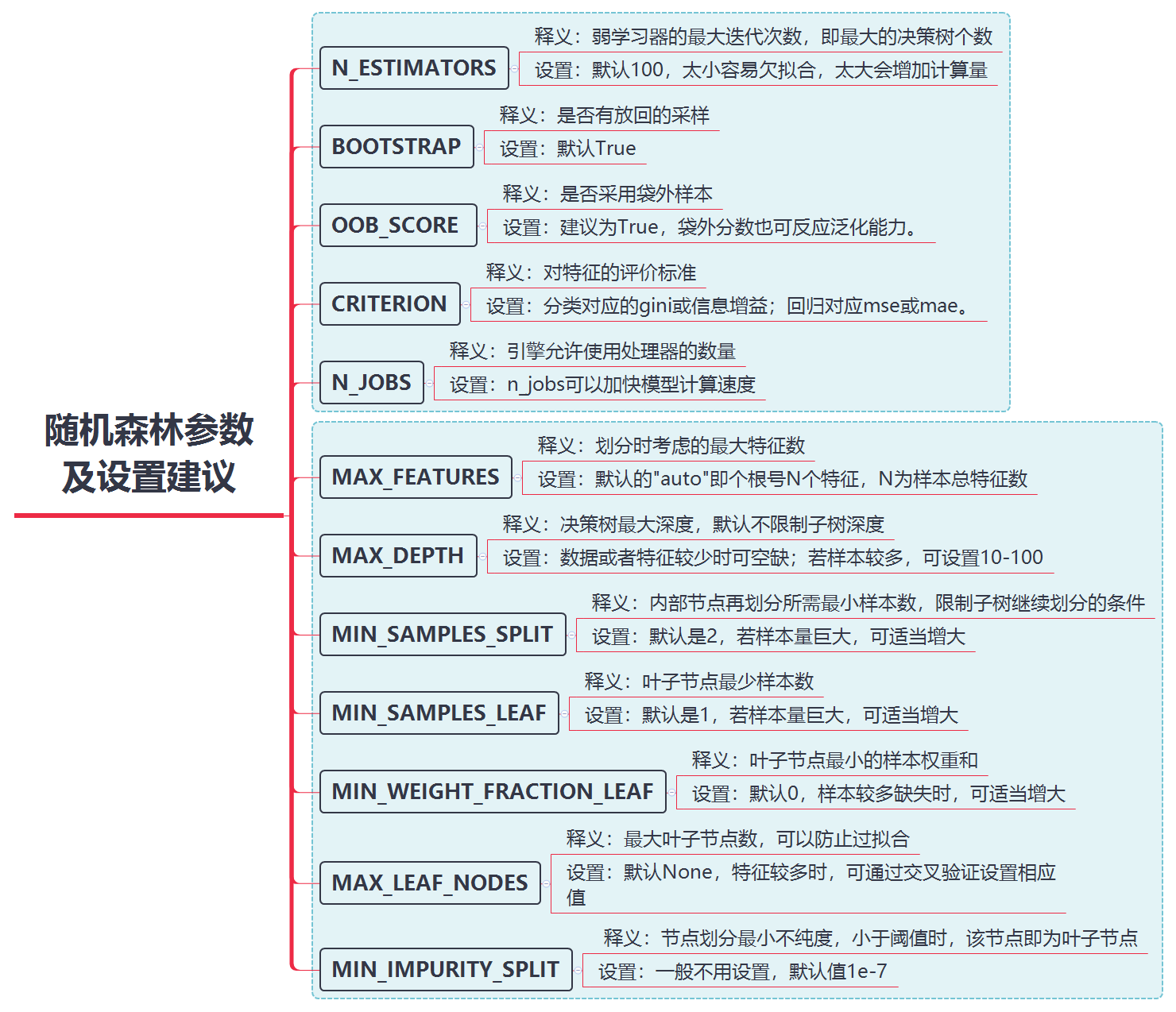其中，bootstrap参数：代表的是bootstrap sample。也就是又放回抽样，bootstrap生成的数据集和原始数据集在数据量上是一致的，但由于进行了重复采样，因此其中有一些数据点会丢失。通过重新生成数据集，可以让随机森林中的每一棵决策树在构建的时候，彼此之间有差异。max_features的取值越高，随机森林中的每一棵树就会"越相似"，从而因为有更多的不同特征值可以取，也就会更容易取拟合数据。
# 定义图像中分区的颜色和散点的颜色
cmap_light = ListedColormap(["#FFAAAA", "#AAFFAA", "#AAAAFF"])
cmap_bold = ListedColormap(["#FF0000", "#00FF00", "#0000FF"])

# 分别用样本的两个特征值创建图像和横轴、纵轴
x_min, x_max = X_train[:,0].min() - 1, X_train[:,0].max() + 1
y_min, y_max = X_train[:,1].min() - 1, X_train[:,1].max() + 1
xx, yy = np.meshgrid(np.arange(x_min, x_max, .02),np.arange(y_min, y_max, .02))
z = forest.predict(np.c_[xx.ravel(), yy.ravel()])
# 给每个分类中的样本分配不同的颜色
z = z.reshape(xx.shape)
plt.figure()
plt.pcolormesh(xx, yy, z, cmap=cmap_light)

# 使用散点图进行表示
plt.scatter(X[:,0], X[:,1], c=y, cmap=cmap_bold, edgecolor="k",s=20)
plt.xlim(xx.min(), xx.max())
plt.ylim(yy.min(), yy.max())
plt.title("Classifier:(max_depth = 3)")
plt.show()随机森林实例
author by xiaoyao
# 导入相应的libraries
import pandas as pd
from sklearn import tree
from sklearn.ensemble import RandomForestClassifier
from sklearn.model_selection import train_test_split
# 忽略警告
import warnings
warnings.filterwarnings("ignore")

# 导入数据集，数据集下载地址：http://archive.ics.uci.edu/ml/machine-learning-databases/adult/
# 原始数据无列名（属性名）
names=['年龄','单位性质','权重','学历','受教育时常',
'婚姻状况','职业','家庭情况','种族','性别',
'资产所得','资产损失','周工作时长','原籍','收入'])


import pandas_profiling
rep = pandas_profiling.ProfileReport(data)

# 将生成的数据分析报告保存至本地html文件
rep.to_file("./rep.html")

报告具体形式: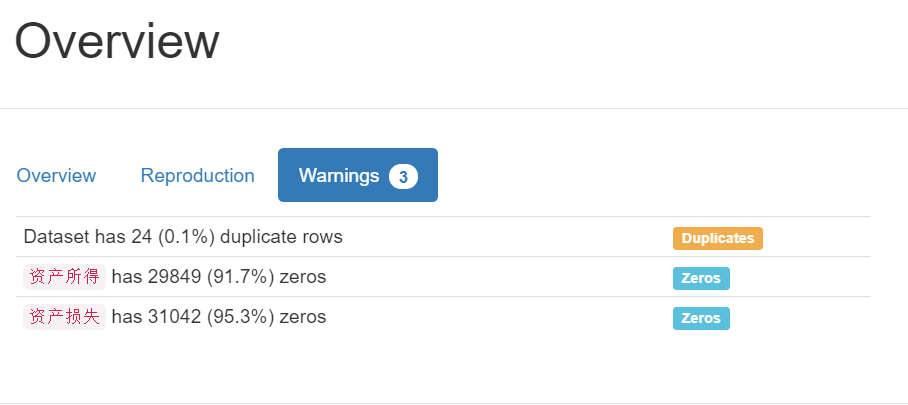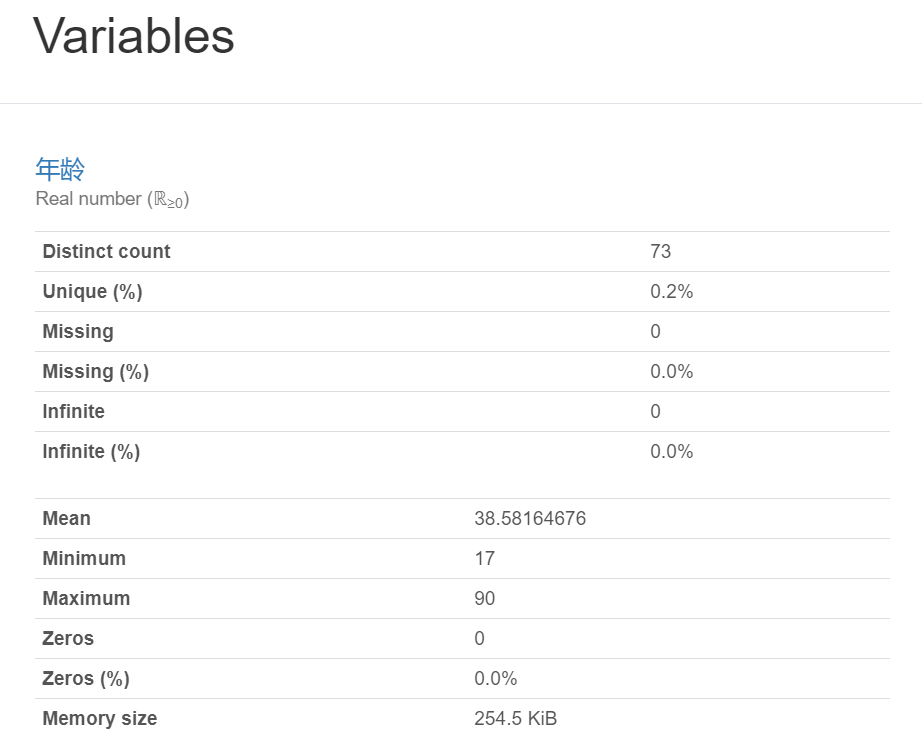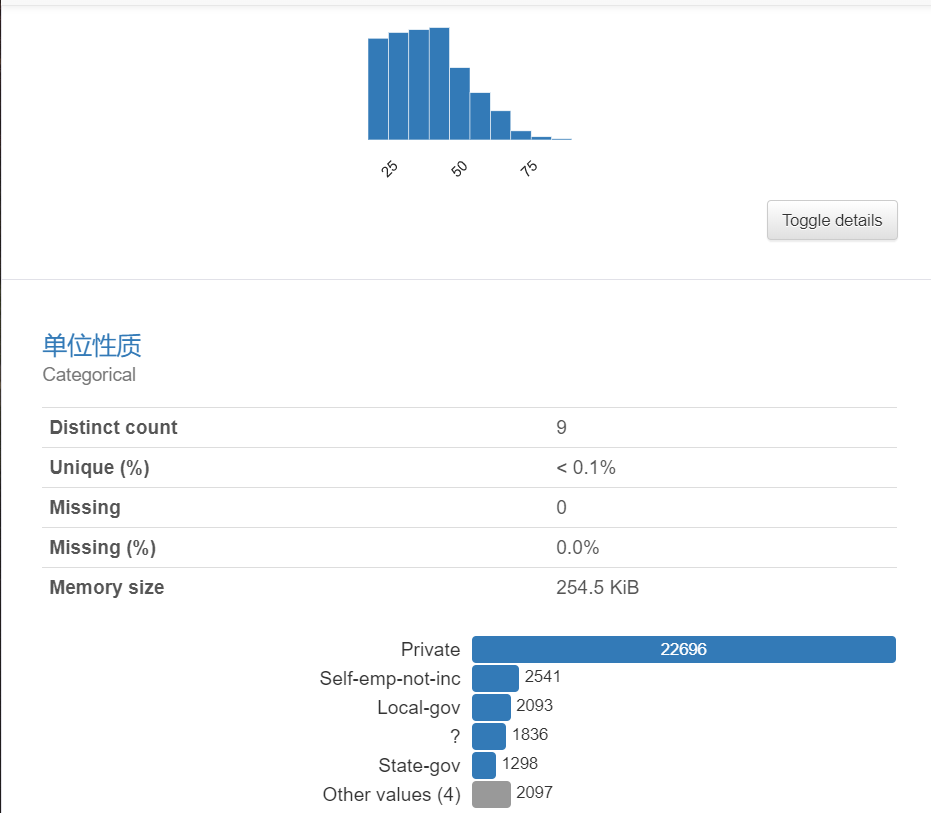⋮

\vdots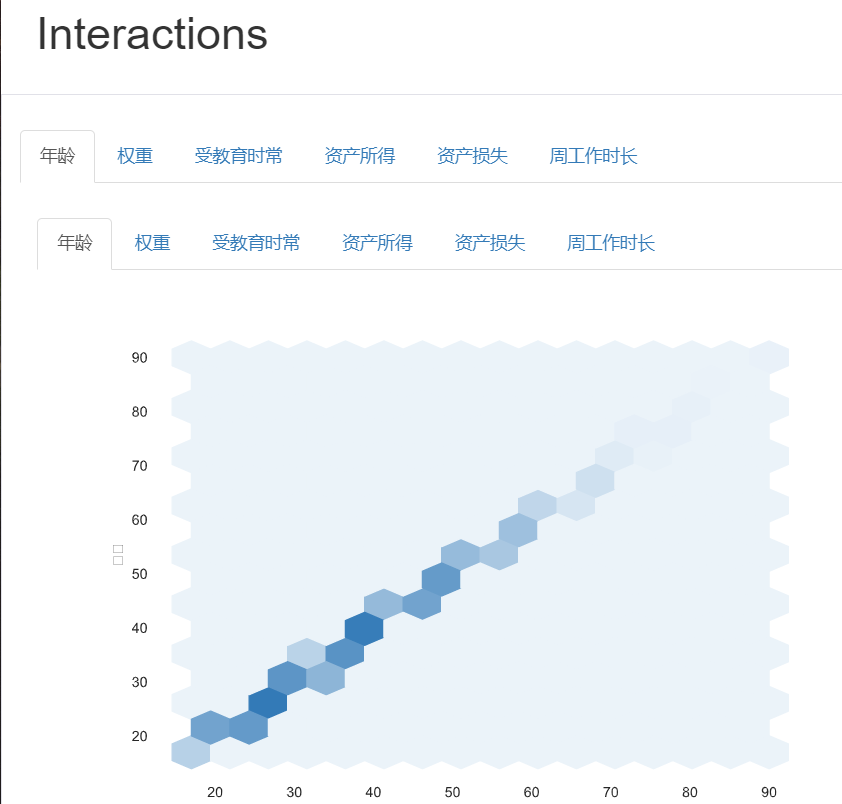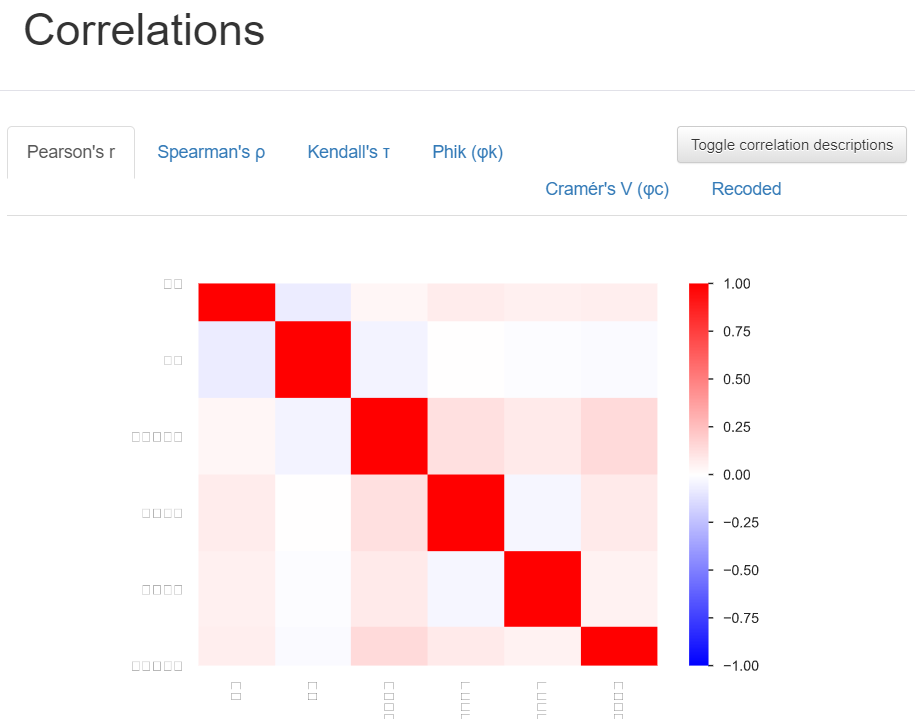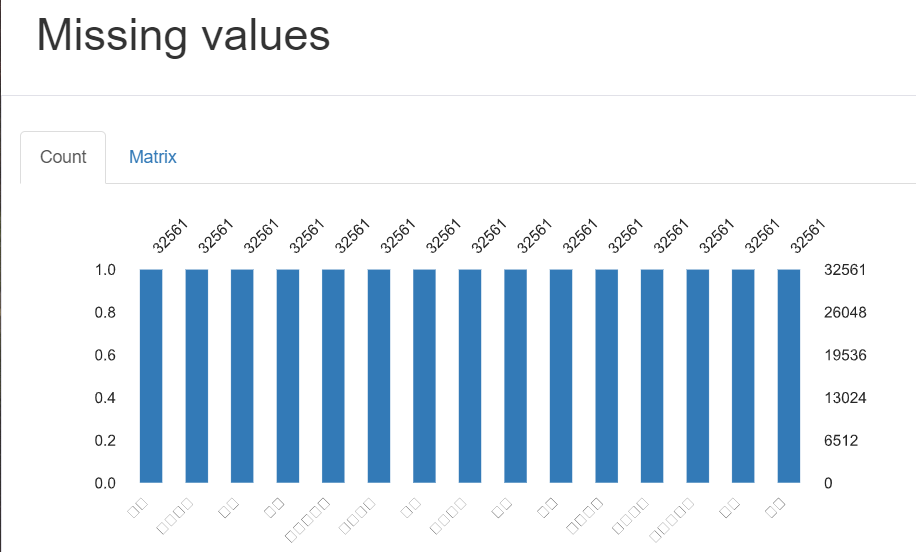data.info()

<class 'pandas.core.frame.DataFrame'>
RangeIndex: 32561 entries, 0 to 32560
Data columns (total 15 columns):
年龄       32561 non-null int64
单位性质     32561 non-null object
权重       32561 non-null int64
学历       32561 non-null object
受教育时常    32561 non-null int64
婚姻状况     32561 non-null object
职业       32561 non-null object
家庭情况     32561 non-null object
种族       32561 non-null object
性别       32561 non-null object
资产所得     32561 non-null int64
资产损失     32561 non-null int64
周工作时长    32561 non-null int64
原籍       32561 non-null object
收入       32561 non-null object
dtypes: int64(6), object(9)
memory usage: 3.7+ MB

可以看到在数据集中，很多的列取值都是字符串，不方便进行建模。我将其处理为：整型数值，操作如下：
# 使用get_dummies将文本数据转化为数值
data_dummies = pd.get_dummies(data)
# 分别输出原始特征和处理之后的特征
print("原始特征:",(data.columns))
print("处理后的特征:",(data_dummies.columns))

原始特征: Index(['年龄', '单位性质', '权重', '学历', '受教育时常', '婚姻状况', '职业', '家庭情况', '种族', '性别',
'资产所得', '资产损失', '周工作时长', '原籍', '收入'],
dtype='object')
处理后的特征: Index(['年龄', '权重', '受教育时常', '资产所得', '资产损失', '周工作时长', '单位性质_ ?',
'单位性质_ Federal-gov', '单位性质_ Local-gov', '单位性质_ Never-worked',
...
'原籍_ Scotland', '原籍_ South', '原籍_ Taiwan', '原籍_ Thailand',
'原籍_ Yugoslavia', '收入_ <=50K', '收入_ >50K'],
dtype='object', length=110)

data.head()


data_dummies.head()


年龄权重受教育时常资产所得资产损失周工作时长单位性质_ ?单位性质_ Federal-gov单位性质_ Local-gov单位性质_ Never-worked...原籍_ Scotland原籍_ South原籍_ Taiwan原籍_ Thailand原籍_ Trinadad&Tobago原籍_ United-States原籍_ Vietnam原籍_ Yugoslavia收入_ <=50K收入_ >50K039775161321740400000...0000010010150833111300130000...0000010010238215646900400000...0000010010353234721700400000...00000100104283384091300400000...0000000010
5 rows × 110 columns
将各列分配给特征向量

X

X

和分类标签

y

y

# 定义数据集的特征值
features = data_dummies.loc[:, '年龄':'原籍_ Yugoslavia']
X = features.values
# 将收入大于50k作为预测的目标
y = data_dummies['收入_ >50K'].values
# 输出特征与标签的形态
print("特征形态:{} 标签形态:{}".format(X.shape, y.shape))

特征形态:(32561, 108) 标签形态:(32561,)

# 先使用决策数模型进行预测
# 将数据拆分为训练集和测试集
X_train, X_test, y_train, y_test = train_test_split(X, y, random_state = 1)
# 设最大深度为5
dt_tree =tree.DecisionTreeClassifier(max_depth=5)
dt_tree.fit(X_train, y_train)
dt_tree.score(X_test, y_test)

0.8550546615894853

# 使用交叉验证,平均水平依然接近上面的
from sklearn.model_selection import cross_val_score
scores = cross_val_score(dt_tree, X, y, cv=20,scoring='accuracy')
print(scores)
print(scores.mean())

[0.83977901 0.84275184 0.8482801  0.84336609 0.85012285 0.8531941
0.82555283 0.84398034 0.8458231  0.85380835 0.85995086 0.83783784
0.85257985 0.84889435 0.86425061 0.85257985 0.8507371  0.86302211
0.84766585 0.84643735]
0.8485307193180122

# 使用随机森林算法
forest = RandomForestClassifier(n_estimators = 5,random_state=0)
forest.fit(X_train, y_train)
forest.score(X_test, y_test)

0.8431396634320109

# 使用交叉验证
scores = cross_val_score(forest, X, y,cv=5,scoring='accuracy')
print(scores)
print(scores.mean())

[0.82320442 0.83968059 0.83230958 0.84336609 0.82862408 0.85012285
0.82678133 0.8458231  0.84336609 0.83108108 0.83722359 0.83046683
0.84336609 0.83599509 0.8482801  0.84213759 0.84766585 0.85257985
0.83169533 0.84459459]
0.8389182062524604

展开全文决策树 机器学习 数据挖掘
• 随机森林就是通过集成学习的思想将多棵树集成的一种算法，它的基本单元是决策树，而它的本质属于机器学习的一大分支——集成学习（Ensemble Learning）方法。随机森林的名称中有两个关键词，一个是“随机”，一个...
1.什么是随机森林？
如果读者接触过决策树（Decision Tree） 的话，那么会很容易理解什么是随机森林。随机森林就是通过集成学习的思想将多棵树集成的一种算法，它的基本单元是决策树，而它的本质属于机器学习的一大分支——集成学习（Ensemble Learning）方法。随机森林的名称中有两个关键词，一个是“随机”，一个就是“森林”。“森林”我们很好理解，一棵叫做树，那么成百上千棵就可以叫做森林了，这样的比喻还是很贴切的，其实这也是随机森林的主要思想–集成思想的体现。“随机”的含义我们会在下边部分讲到。
其实从直观角度来解释，每棵决策树都是一个分类器（假设现在针对的是分类问题），那么对于一个输入样本，N棵树会有N个分类结果。而随机森林集成了所有的分类投票结果，将投票次数最多的类别指定为最终的输出，这就是一种最简单的 Bagging 思想。
Each tree is grown as follows:
If the number of cases in the training set is N, sample N cases at random - but with replacement, from the original data. This sample will be the training set for growing the tree.If there are M input variables, a number m<<M is specified such that at each node, m variables are selected at random out of the M and the best split on these m is used to split the node. The value of m is held constant during the forest growing.Each tree is grown to the largest extent possible. There is no pruning.
In the original paper on random forests, it was shown that the forest error rate depends on two things:
The correlation between any two trees in the forest. Increasing the correlation increases the forest error rate.The strength of each individual tree in the forest. A tree with a low error rate is a strong classifier. Increasing the strength of the individual trees decreases the forest error rate.
2 随机森林的特点
我们前边提到，随机森林是一种很灵活实用的方法，它有如下几个特点：
在当前所有算法中，具有极好的准确率/It is unexcelled in accuracy among current algorithms；能够有效地运行在大数据集上/It runs efficiently on large data bases；能够处理具有高维特征的输入样本，而且不需要降维/It can handle thousands of input variables without variable deletion；能够评估各个特征在分类问题上的重要性/It gives estimates of what variables are important in the classification；在生成过程中，能够获取到内部生成误差的一种无偏估计/It generates an internal unbiased estimate of the generalization error as the forest building progresses；对于缺省值问题也能够获得很好得结果/It has an effective method for estimating missing data and maintains accuracy when a large proportion of the data are missingIt has methods for balancing error in class population unbalanced data sets.Generated forests can be saved for future use on other data.Prototypes are computed that give information about the relation between the variables and the classification.It computes proximities between pairs of cases that can be used in clustering, locating outliers, or (by scaling) give interesting views of the data.
实际上，随机森林的特点不只有这六点，它就相当于机器学习领域的Leatherman（多面手），你几乎可以把任何东西扔进去，它基本上都是可供使用的。在估计推断映射方面特别好用，以致都不需要像SVM那样做很多参数的调试。
3 随机森林的相关基础知识
1) 信息、熵以及信息增益的概念
这三个基本概念是决策树的根本，是决策树利用特征来分类时，确定特征选取顺序的依据。理解了它们，决策树你也就了解了大概。
信息 的概念：信息是对一个抽象事物的命名，无论用不用‘信息’来命名这种抽象事物，或者用其他名称来命名这种抽象事物，这种抽象事物是客观存在的。 引用香农的话来说，信息是用来消除随机不确定性的东西。 当然这句话虽然经典，但是还是很难去搞明白这种东西到底是个什么样，可能在不同的地方来说，指的东西又不一样。  熵的概念 熵是约翰.冯.诺依曼建议使用的命名（当然是英文），在信息论和概率论中熵是对随机变量不确定性的度量,与上边联系起来，熵便是信息的期望值。 熵只依赖X的分布，和X的取值没有关系，熵是用来度量不确定性，当熵越大，概率为X=xi的不确定性越大，反之越小，在机器学习分类中，熵越大即这个类别的不确定性更大，反之越小。 当p=0或p=1时，H§=0,随机变量完全没有不确定性，当p=0.5时，H§=1,此时随机变量的不确定性最大 条件熵 条件熵是用来解释信息增益而引入的概念，概率定义：随机变量X在给定条件下随机变量Y的条件熵，对定义描述为：X给定条件下Y的条件概率分布的熵对X的数学期望，在机器学习中为选定某个特征后的熵。  信息增益 信息增益在决策树算法中是用来选择特征的指标，信息增益越大，则这个特征的选择性越好，在概率中定义为：待分类的集合的熵和选定某个特征的条件熵之差（这里指的是经验熵或经验条件熵，由于真正的熵并不知道，是根据样本计算出来的） 注意：这里不要理解偏差，因为上边说了熵是类别的，但是在这里又说是集合的熵，没区别，因为在计算熵的时候是根据各个类别对应的值求期望来等到熵
2)决策树
决策树是一种树形结构，其中每个内部节点表示一个属性上的测试，每个分支代表一个测试输出，每个叶节点代表一种类别。常见的决策树算法有C4.5、ID3和CART。
3)集成学习
集成学习通过建立几个模型组合的来解决单一预测问题。它的工作原理是生成多个分类器/模型，各自独立地学习和作出预测。这些预测最后结合成单预测，因此优于任何一个单分类的做出预测。 ​ 随机森林是集成学习的一个子类，它依靠于决策树的投票选择来决定最后的分类结果。你可以在这找到用python实现集成学习的文档：Scikit 学习文档。https://scikit-learn.org/dev/modules/ensemble.html
4. python实现
class sklearn.ensemble.RandomForestClassifier(n_estimators=10, criterion='gini', max_depth=None, min_samples_split=2, min_samples_leaf=1, min_weight_fraction_leaf=0.0, max_features=’auto’, max_leaf_nodes=None, min_impurity_decrease=0.0, min_impurity_split=None, bootstrap=True, oob_score=False, n_jobs=1, random_state=None, verbose=0, warm_start=False, class_weight=None)

A random forest is a meta estimator that fits a number of decision tree classifiers on various sub-samples of the dataset and uses averaging to improve the predictive accuracy and control over-fitting. The sub-sample size is controlled with the max_samples parameter if bootstrap=True (default), otherwise the whole dataset is used to build each tree.
Parameters:
n_estimators:int, default=100 The number of trees in the forest.criterion:{“gini”, “entropy”}, default=”gini” The function to measure the quality of a split. Supported criteria are “gini” for the Gini impurity and “entropy” for the information gain. Note: this parameter is tree-specific.max_depth:int, default=None The maximum depth of the tree. If None, then nodes are expanded until all leaves are pure or until all leaves contain less than min_samples_split samples.min_samples_split:int or float, default=2 The minimum number of samples required to split an internal node:
If int, then consider min_samples_split as the minimum number.If float, then min_samples_split is a fraction and ceil(min_samples_split * n_samples) are the minimum number of samples for each split. min_samples_leaf:int or float, default=1 The minimum number of samples required to be at a leaf node. A split point at any depth will only be considered if it leaves at least min_samples_leaf training samples in each of the left and right branches. This may have the effect of smoothing the model, especially in regression.
"""
RandomForestClassifier
skleran 的随机森林回归模型，应用流程。
2.源数据随机的切分：75%作为训练数据  25%最为测试数据
1.训练数据中的因变量（分类变量）处理成数字形式
3.设定参数，训练/fit
4.对测试数据，预测/predict结果y_pre
5.对预测数据y列,y_pre列,生成混淆矩阵,显示分类/预测效果
"""

from sklearn.ensemble import RandomForestClassifier
import pandas as pd
import numpy as np

#读取鸢尾花数据集
#DataFrame(数据，columns列名 index行名)
df = pd.DataFrame(iris.data, columns=iris.feature_names)
#numpy.random.uniform(low,high,size)
#功能：从一个均匀分布[low,high)中随机采样，注意定义域是左闭右开，即包含low，不包含high.
#判断：小于0.75的判为true
df['is_train'] = np.random.uniform(0, 1, len(df)) <= .75 #相当于随机抽取了75%作为训练数据
#将数字类别转为文字类别
df['species'] = pd.Categorical.from_codes(iris.target, iris.target_names)

train, test = df[df['is_train']==True], df[df['is_train']==False]#拆分训练集和测试集

features = df.columns[:4]  # 前4个指标 为自变量
clf = RandomForestClassifier(n_jobs=-1)  # n_jobs=2是线程数   n_jobs调为-1表示 cpu有多少核，就有多少并行数
y, _ = pd.factorize(train['species'])# 将文字类别 转为数字类别。一种序列化方法。
# 第一参数是序列化后结果，第二个是参考
clf.fit(train[features], y)          # 训练过程
print(clf.decision_path(train[features]))
preds = iris.target_names[clf.predict(test[features])] # 获取测试数据预测结果
pd.crosstab(test['species'], preds, rownames=['actual'], colnames=['preds'])#生成混淆矩阵

import numpy as np
import matplotlib.pyplot as plt
from matplotlib.colors import ListedColormap
from sklearn.model_selection import train_test_split
from sklearn.preprocessing import StandardScaler
from sklearn.datasets import make_moons, make_circles, make_classification
from sklearn.neighbors import KNeighborsClassifier
from sklearn.svm import SVC
from sklearn.tree import DecisionTreeClassifier
from sklearn.naive_bayes import GaussianNB
#from sklearn.lda import LDA
from sklearn.discriminant_analysis import LinearDiscriminantAnalysis as LDA
from sklearn.discriminant_analysis import QuadraticDiscriminantAnalysis as QDA

h = .02  # step size in the mesh

names = ["Nearest Neighbors", "Linear SVM", "RBF SVM", "Decision Tree",
"Random Forest", "AdaBoost", "Naive Bayes", "LDA", "QDA"]
classifiers = [
KNeighborsClassifier(3),
SVC(kernel="linear", C=0.025),
SVC(gamma=2, C=1),
DecisionTreeClassifier(max_depth=5),
RandomForestClassifier(max_depth=5, n_estimators=10, max_features=1),
GaussianNB(),
LDA(),
QDA()]

X, y = make_classification(n_features=2, n_redundant=0, n_informative=2,
random_state=1, n_clusters_per_class=1)
rng = np.random.RandomState(2)
X += 2 * rng.uniform(size=X.shape)
linearly_separable = (X, y)

datasets = [make_moons(noise=0.3, random_state=0),
make_circles(noise=0.2, factor=0.5, random_state=1),
linearly_separable
]

figure = plt.figure(figsize=(27, 9))
i = 1
# iterate over datasets
for ds in datasets:
# preprocess dataset, split into training and test part
X, y = ds
X = StandardScaler().fit_transform(X)
X_train, X_test, y_train, y_test = train_test_split(X, y, test_size=.4)

x_min, x_max = X[:, 0].min() - .5, X[:, 0].max() + .5
y_min, y_max = X[:, 1].min() - .5, X[:, 1].max() + .5
xx, yy = np.meshgrid(np.arange(x_min, x_max, h),
np.arange(y_min, y_max, h))

# just plot the dataset first
cm = plt.cm.RdBu
cm_bright = ListedColormap(['#FF0000', '#0000FF'])
ax = plt.subplot(len(datasets), len(classifiers) + 1, i)
# Plot the training points
ax.scatter(X_train[:, 0], X_train[:, 1], c=y_train, cmap=cm_bright)
# and testing points
ax.scatter(X_test[:, 0], X_test[:, 1], c=y_test, cmap=cm_bright, alpha=0.6)
ax.set_xlim(xx.min(), xx.max())
ax.set_ylim(yy.min(), yy.max())
ax.set_xticks(())
ax.set_yticks(())
i += 1

# iterate over classifiers
for name, clf in zip(names, classifiers):
ax = plt.subplot(len(datasets), len(classifiers) + 1, i)
clf.fit(X_train, y_train)
score = clf.score(X_test, y_test)

# Plot the decision boundary. For that, we will assign a color to each
# point in the mesh [x_min, m_max]x[y_min, y_max].
if hasattr(clf, "decision_function"):
Z = clf.decision_function(np.c_[xx.ravel(), yy.ravel()])
else:
Z = clf.predict_proba(np.c_[xx.ravel(), yy.ravel()])[:, 1]

# Put the result into a color plot
Z = Z.reshape(xx.shape)
ax.contourf(xx, yy, Z, cmap=cm, alpha=.8)

# Plot also the training points
ax.scatter(X_train[:, 0], X_train[:, 1], c=y_train, cmap=cm_bright)
# and testing points
ax.scatter(X_test[:, 0], X_test[:, 1], c=y_test, cmap=cm_bright,
alpha=0.6)

ax.set_xlim(xx.min(), xx.max())
ax.set_ylim(yy.min(), yy.max())
ax.set_xticks(())
ax.set_yticks(())
ax.set_title(name)
ax.text(xx.max() - .3, yy.min() + .3, ('%.2f' % score).lstrip('0'),
size=15, horizontalalignment='right')
i += 1

plt.show()

展开全文• 点击上方“Python爬虫与数据挖掘”，进行关注回复“书籍”即可获赠Python从入门到进阶共10本电子书今日鸡汤寒梅最堪恨，长作去年花。前言组合算法也叫集成学习，在金融行业或非图像识别...

点击上方“Python爬虫与数据挖掘”，进行关注
回复“书籍”即可获赠Python从入门到进阶共10本电子书
今
日
鸡
汤
寒梅最堪恨，长作去年花。
前言
组合算法也叫集成学习，在金融行业或非图像识别领域，效果有时甚至比深度学习还要好。能够理解基本原理并将代码用于实际的业务案例是本文的目标，本文将详细介绍如何利用Python实现集成学习中随机森林这个经典的方法来预测宽带客户的流失，主要将分为两个部分：
详细原理介绍Python代码实战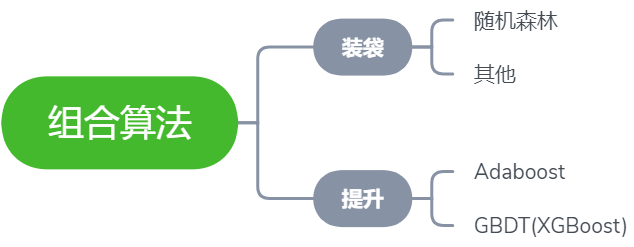集成学习
本文的主角是随机森林，所以我们将以随机森林所属的分支 —— 装袋法 入手，深入浅出该集成学习方法的原理步骤。装袋法流程如下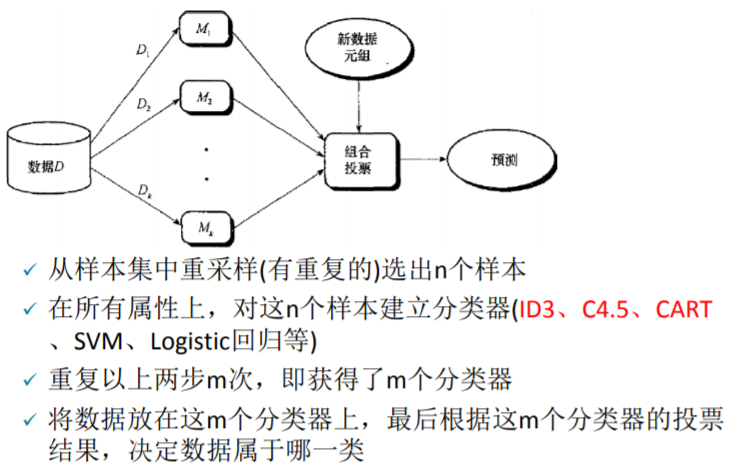乍一看图中的步骤可能有些复杂，现在来逐步拆解。装袋法中的装袋二字是精髓，顾名思义即将多个模型装入同一个袋子后，让这个袋子作为一个新的模型来实现预测需求，仅此而已。换句话说，即把多个模型组合起来形成一个新的大模型，这个大模型最终给出的预测结果是由这多个小模型综合决定的，决定方式为少数服从多数。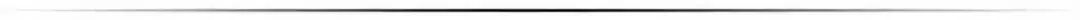假设有10万条原始数据，用这些数据来做十棵决策树（当然也可以是其他模型），最后这10棵树将被装进了同一个袋子中。这时候取其中一条数据放入这个袋子，便会得出10个预测值(每棵树各一个)，假如其中三棵树给出的预测值为0，剩余的七棵给出的为1，那我们便可知道这个袋子对这个数据的预测结果为 0 的概率是 3/10。为了更深入的理解装袋法，下面将回答三个与装袋法有关的常见问题：
问：袋子中的每个模型使用的样本量范围应为多少合适？
答：如果是上面的例子，袋子里面有十棵树，源数据总量为 10万 条，则每棵树取用的样本量的最小值为最少是1w个(10w/10棵 = 1w/棵)，因为至少要保证不能浪费样本，但每棵树最多可取用多少样本呢？其实在样本量已知，同一袋子中模型个数为n的情况下，样本的选择比例为1/n ~ 0.8最好。每个小模型取用 100% 的样本是绝对没有意义的，那就跟没抽是一样的，这样也就没有体现出装袋，只有每个模型用到的数据都有一定的不同，组合起来后每个的投票（预测结果）也才有意义。问：袋中模型们之间的相关性会影响最后的决策结果吗？
答：装袋法思路最重要的一点：袋子中每个模型之间不能相关，越不相关越好，这里的不相关主要体现在用于训练每个模型的样本不一样。其次，每个模型的精度越高越好，这样它的投票才更有价值。
PS：训练模型的样本不一样这一点可以理解为总统选举，抽 10 波选民来投票，这 10 波选民的差异性越大越好，这样一来，只有在选民千差万别的情况下你依然脱颖而出，才足以说明你的实力，如果这10波选民中每一波之间的差异性都很小，比如都是本来就偏袒于总统候选人，那投票结果的说服力就会大减。问：上面所说的模型精度高是不是哪怕模型很复杂也可以，如果每个模型的精度高但都过度拟合怎么办？
答：在装袋法中，模型是越精确越好，哪怕是过度拟合的也没有关系。因为一个模型要想在训练集上做到越精确越好，而精确程度与模型的复杂度大多是成正比的，所以出现过拟合的情况也是正常且情有可原的。复杂和过度拟合只是对袋子中每个模型而言，因为最后都会被加权，所以整个袋子（整体）并不会出现过度拟合的情况。
随机森林
随机森林的实现步骤如下：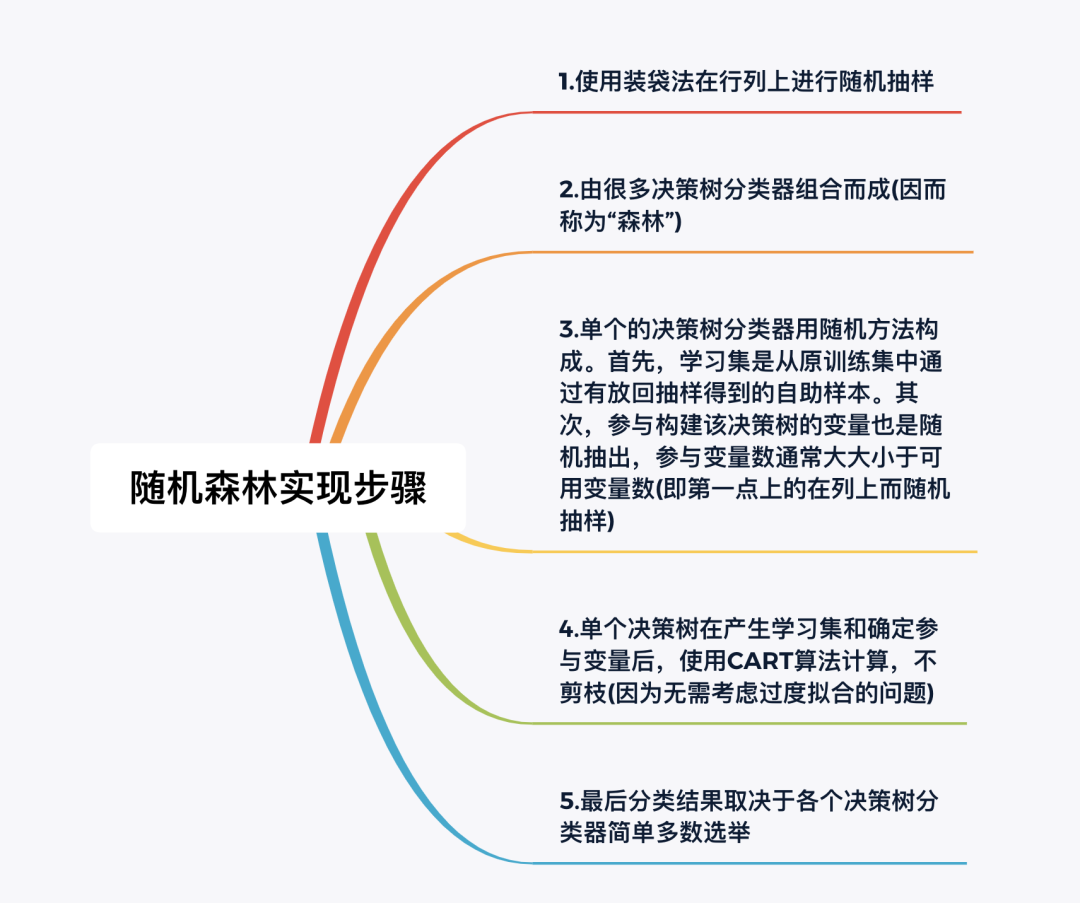有关随机森林算法，本文说明以下几个问题
问：为什么在列上也要随机抽样？
答：在引入笔者最最喜欢的一个比喻之前，先来看一个实际的业务场景，来自某城市商业银行。我们有一大个电子表格存着大量的历史数据，大概50多个变量(50多列)，变量们来自几个不同的公司如人行，电信等(同一个客户在不同公司)，最后希望预测的是该客户是否会违约。电子表格组成如下：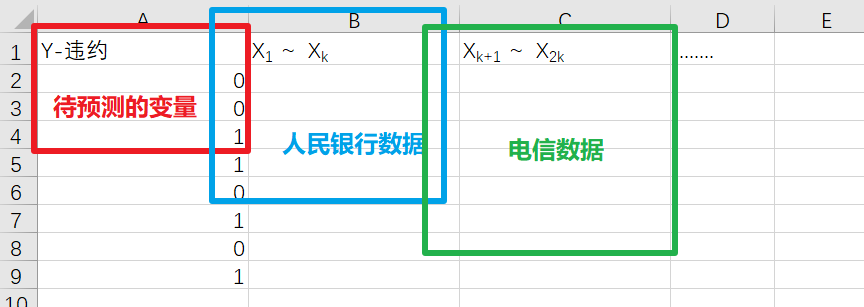而根据基础的业务知识可知，与银行有关的数据中往往会存在许多缺失值，以上图为例，通常情况下只有待预测的变量这一列的数据是齐全的，毕竟客户们是否违约这个行为的历史数据很容易查找，但蓝框和绿框这两部分的缺失值往往较多，而且较随意，具体随意程度参见下图：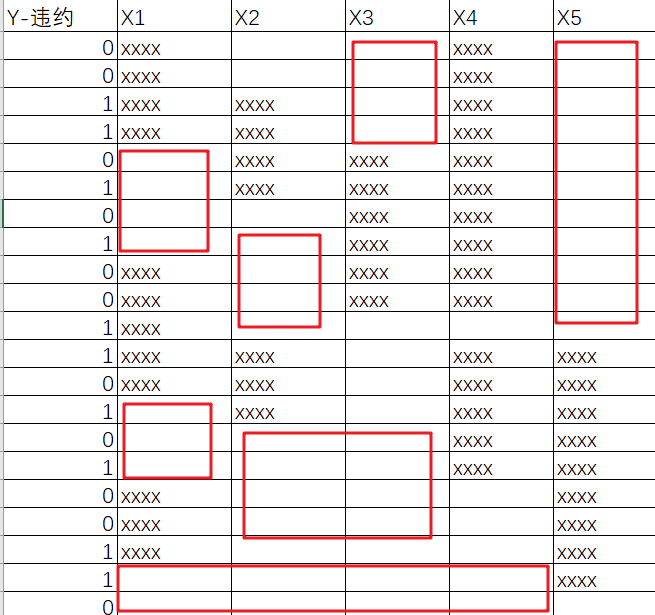红框表示数据缺失，这里只展示了部分行和部分列数据，如果这份数据表的规模为 4万行 * 50列，那这数据缺失的分布得有多随意啊 ????？？所以，到底该如何充分利用这残次不齐的数据就成了呈待解决的关键问题。这时候就可以祭出超级生动形象的 “岛屿 - 湖泊 - 椰子树”比喻了：
整个表格看成一座巨大的岛屿，岛屿的长和宽分别对应电子表格横轴长和纵轴的长度表中缺失的数据段看成一个个分布随意的小湖泊，有数据的地方看成陆地整个小岛地底埋藏着巨大的价值（数据价值），通过在随意的种树（用装袋法在行列上进行随机抽样）来吸取地底的养分，毕竟湖泊上种不了树，所以只要足够随机，就总能充分的利用陆地。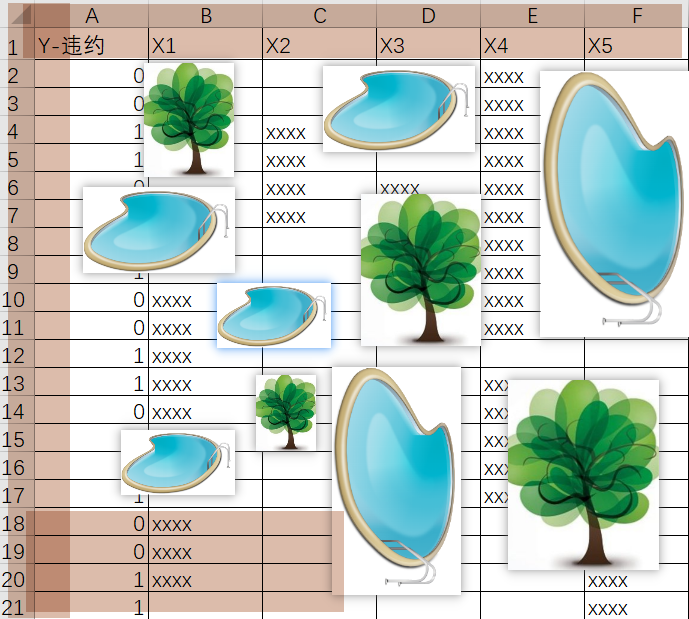正因为是行列都随机，才能够做到真正的把整个数据表随机切分成多份，每个模型使用一份，只要模型的数量足够，总有模型能够在最大程度上获取数据集的价值。而且因变量的分类往往又是极不平衡的，可以参考原理+代码｜手把手教你使用Python实战反欺诈模型。至于如何将这些种好的树的信息又再收集，便可以将陆地上比较近的几棵树上面再弄一个收集器，把这几棵树从陆地上收集到的养分再递进一层汇总，最终实现陆地养分汇总于树木，树木养分汇总于收集器，收集器养分汇总于更上层的另一个收集器，最终实现整片数据海洋中多个岛屿的信息汇总，这便是周志华团队和蚂蚁金服的合作的用分布式深度随机森林算法检测套现欺诈。
随机森林第一步之后的操作完全可以参照集成学习——装袋法中提及的步骤。问：既然每个模型给出的预测结果最后都会被加权，所以随机森林中每棵决策树的权重是多少？问：装袋法中袋子中的模型越多越好吗？袋中用来训练每个模型的源数据比例也是越多越好吗？
答：袋子中模型多一点好，袋中用来训练每个模型的源数据比例小一点好，但这并不代表越多越好与越小越好，还得结合数据集特性和一些深层次的模型算法知识。
装袋法的优势如下：
准确率明显高于组合中任何单个分类器对于较大的噪音，表现不至于很差，并且具有鲁棒性不容易过度拟合
随机森林算法的优点：
准确率有时可以和神经网络媳美，比逻辑回归高对错误和离群点更加鲁棒性决策树容易过度拟合的问题会随着森林的规模而削弱大数据情况下速度快(分布式)，性能好
Python实战
数据探索
本次实战目标为演示随机森林的用法和调优方法。因为集成学习与神经网络一样，都属于解释性较差的黑盒模型，所以我们无需过分探究数据集中每个变量的具体含义，只需关注最后一个变量broadband即可，争取通过如年龄，使用时长，支付情况以及流量和通话情况等变量对宽带客户是否会续费做出一个较准确的预测。
import pandas as pd
import numpy as np

df.head(); df.info()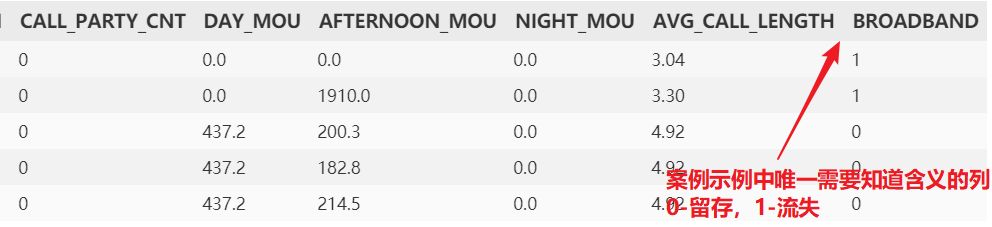参数说明
本代码文件只为演示随机森林的用法和调优方法，所以数据参数我们只需关注最后一个broadband 即可0-离开，1-留存。其他自变量意思可不做探究，毕竟真实工作中的数据集也完全不一样，首先将列名全部小写
df.rename(str.lower, axis='columns', inplace=True)
现在查看因变量broadband分布情况，看是否存在不平衡
from collections import Counter
## Broadband:  Counter({0: 908, 1: 206}) 比较不平衡。
## 根据原理部分，可知随机森林是处理数据不平衡问题的利器
接着拆分测试集与训练集，客户id没有用，故丢弃cust_id,
y = df['broadband']
X = df.iloc[:, 1:-1]
from sklearn.model_selection import train_test_split
X_train, X_test, y_train, y_test = train_test_split(X, y,
test_size=0.4, random_state=12345)
决策树建模
我们先进行完整的决策树建模来和随机森林进行对比
import sklearn.tree as tree

# 直接使用交叉网格搜索来优化决策树模型，边训练边优化
from sklearn.model_selection import GridSearchCV
# 网格搜索的参数：正常决策树建模中的参数 - 评估指标，树的深度，
## 最小拆分的叶子样本数与树的深度
param_grid = {'criterion': ['entropy', 'gini'],
'max_depth': [2, 3, 4, 5, 6, 7, 8],
'min_samples_split': [4, 8, 12, 16, 20, 24, 28]}
# 通常来说，十几层的树已经是比较深了

clf = tree.DecisionTreeClassifier()  # 定义一棵树
clfcv = GridSearchCV(estimator=clf, param_grid=param_grid,
scoring='roc_auc', cv=4)
# 传入模型，网格搜索的参数，评估指标，cv交叉验证的次数
## 这里也只是定义，还没有开始训练模型

clfcv.fit(X=X_train, y=y_train)

# 使用模型来对测试集进行预测
test_est = clfcv.predict(X_test)

# 模型评估
import sklearn.metrics as metrics

print("决策树准确度:")
print(metrics.classification_report(y_test,test_est))
# 该矩阵表格其实作用不大
print("决策树 AUC:")
fpr_test, tpr_test, th_test = metrics.roc_curve(y_test, test_est)
print('AUC = %.4f' %metrics.auc(fpr_test, tpr_test))

AUC 大于0.5是最基本的要求，可见模型精度还是比较糟糕的，决策树的调优技巧就不再过多展开，我们将在随机森林调优部分展示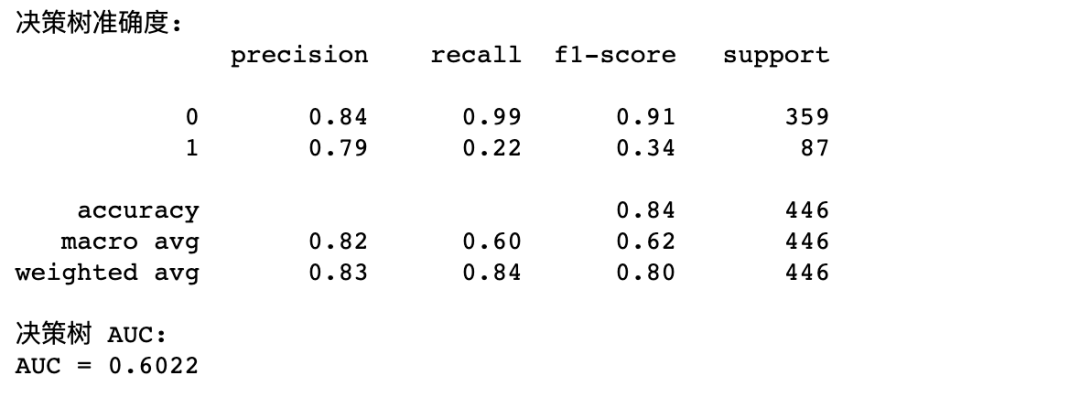随机森林建模
随机森林建模一样是使用网格搜索，有关Python实现随机森林建模的详细参数解释可以看代码的注释
param_grid = {
'criterion':['entropy','gini'],
'max_depth':[5, 6, 7, 8],    # 深度：这里是森林中每棵决策树的深度
'n_estimators':[11,13,15],  # 决策树个数-随机森林特有参数
'max_features':[0.3,0.4,0.5],
# 每棵决策树使用的变量占比-随机森林特有参数（结合原理）
'min_samples_split':[4,8,12,16]  # 叶子的最小拆分样本量
}

import sklearn.ensemble as ensemble # ensemble learning: 集成学习

rfc = ensemble.RandomForestClassifier()
rfc_cv = GridSearchCV(estimator=rfc, param_grid=param_grid,
scoring='roc_auc', cv=4)
rfc_cv.fit(X_train, y_train)

# 使用随机森林对测试集进行预测
test_est = rfc_cv.predict(X_test)
print('随机森林精确度...')
print(metrics.classification_report(test_est, y_test))
print('随机森林 AUC...')
fpr_test, tpr_test, th_test = metrics.roc_curve(test_est, y_test)
# 构造 roc 曲线
print('AUC = %.4f' %metrics.auc(fpr_test, tpr_test))
可以看到，模型的精度大大提升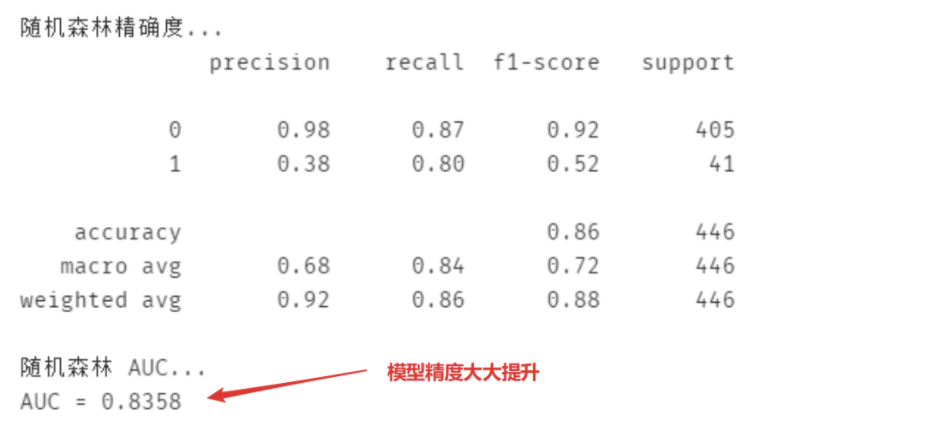为什么要打印梯度优化给出的最佳参数？打印梯度优化结果的最佳参数的目的是为了判断这个分类模型的各种参数是否在决策边界上，简言之，我们不希望决策边界限制了这个模型的效果。（通常这时候会先把复杂度放一边）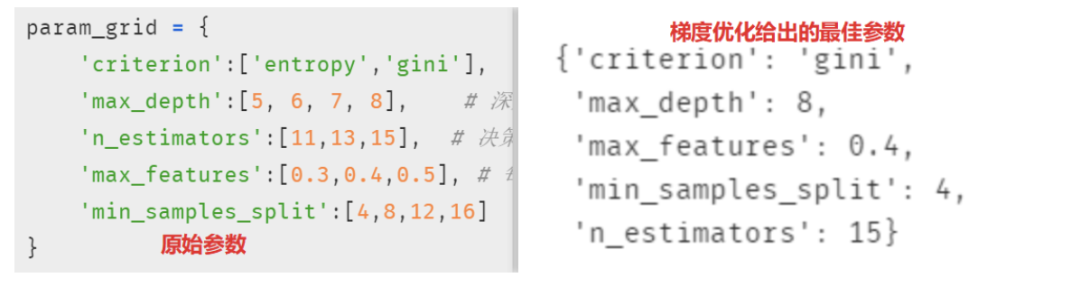不难发现，参数max_depth, min_samples_split, 和n_estimators 这三个参数的范围设置可能有限制模型精度的可能，所以需要适当调整
"""
{'criterion': 'gini',
'max_depth': 8,  在最大值边界上，所以这个参数的最大值范围应该再调大
'max_features': 0.5,  也在最大值边界上，说明这个参数的最小值范围应该再调大
'min_samples_split': 4, 同理，在最小边界上，可考虑把范围调小
'n_estimators': 15 同理，在最大边界上，可以适当调大范围
"""
# 调整结果
param_grid = {
'criterion':['entropy','gini'],
'max_depth':[7, 8, 10, 12],
# 前面的 5，6 也可以适当的去掉，反正已经没有用了
'n_estimators':[11, 13, 15, 17, 19],  #决策树个数-随机森林特有参数
'max_features':[0.4, 0.5, 0.6, 0.7],
#每棵决策树使用的变量占比-随机森林特有参数
'min_samples_split':[2, 3, 4, 8, 12, 16]  # 叶子的最小拆分样本量
现在来查看再次建模的结果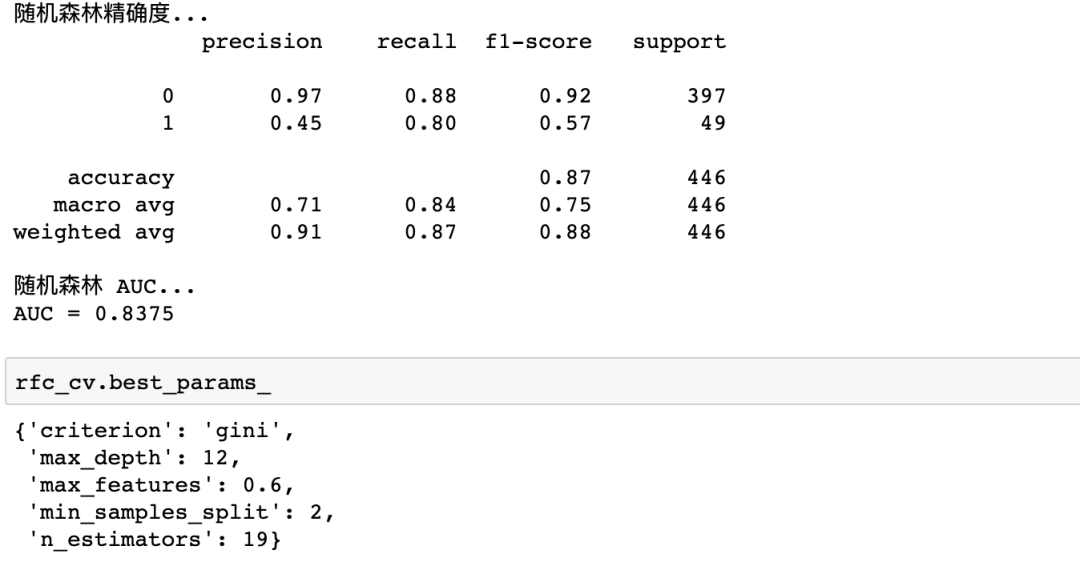此时都在决策边界内了，但其实调整参数是门技术活，并不只是通过决策边界这一单一指标来调整，后续推文会陆续更新。
小结
最后总结一下：随机森林是集成学习中非常经典的一种方法，基础原理简单，实现优雅，可即学即用。而且随机森林应用十分广泛，并不只是局限于常见的金融领域，只要数据不平衡或者随机缺失严重，都值得尝试。
------------------- End -------------------
往期精彩文章推荐：
手把手用Python教你如何发现隐藏wifi手把手教你用Python做个可视化的“剪刀石头布”小游戏Python基础语法——代码规范&判断语句&循环语句欢迎大家点赞，留言，转发，转载，感谢大家的相伴与支持
想加入Python学习群请在后台回复【入群】
万水千山总是情，点个【在看】行不行
/今日留言主题/
随便说一两句吧~~

展开全文决策树 twitter 机器学习 gwt 数据挖掘
• 随机回归森林是由多个完全独立的回归决策树voting完成的，单个决策树的训练采用的是bagging，是集成学习中比较经典，效果较好的方法，可以作为baseline. from sklearn.datasets import load_iris import numpy as ...机器学习 决策树 回归
• 竞赛时写的一个python小程序，得了0.93分，思路如下，1读取训练集、2数据预处理、3上模型、4将模型应用到预测及、5生成预测结果
• 随机森林实现泰坦尼克号数据集的分类预测，包含参数调试过程和分类结果评估，并绘制ROC曲线。
• 定义：随机森林指的是利用多棵决策树对样本进行训练并预测的一种分类器。可回归可分类。所以随机森林是基于多颗决策树的一种集成学习算法，常见的决策树算法主要有以下几种：1. ID3：使用信息增益g(D,A)进行特征选择...
• 回归随机森林算法是用MSE准则来培育每棵决策树，并用决策树平均预测值来计算预测的目标变量。 from sklearn.ensemble import RandomForestRegressor import numpy as np import pandas as pd from sklearn.mode机器学习
• 完全可编译通过，python3代码实现，不调库，纯手撸，带数据集。
• 预测股票市场价格 预测股票价格-线性回归vs随机森林对于这个项目，我使用了包含指数价格的csv文件。 文件中的每一行都包含1950年至2015年间S＆P500... 我将使用该数据集来开发利用线性回归和随机森林算法预测模型。
• 随机森林 kaggle 数据挖掘 categories: 数据挖掘 mathjax: true 文章目录前言：1 数据预处理1.1 读入数据1.2 训练集与数据集1.2.1 查看数据完整性1.2.2 查看训练数据描述信息1.3.1 年龄数据简化分组2 数据可视化2.1...
• ## 随机森林算法Matlab实现

万次阅读 多人点赞 2019-05-25 18:26:32
随机森林算法Matlab实现随机森林算法Matlab实现计算当前自身gini系数求最优划分点及其gini系数对data中按decision属性值从小到大排列生成结点生成随机采样样本数据生成决策树评价函数随机森林样本决策函数正确率计算...二分类 Matlab
• 随机森林顾名思义，是用随机的方式建立一个森林，森林里面有很多的决策树组成，随机森林的每一棵决策树之间是没有关联的。在得到森林之后，当有一个新的输入样本进入的时候，就让森林中的每一棵决策树分别进行一下...
•数据挖掘 kaggle
• 本文拟采用随机森林实现空气质量的预测。 实现环境：python3.5 所需包：pandas 、numpy、matplotlib、csv等。引入包如下： import pandas as pd import numpy as np import matplotlib.pyplot as plt import ...
• ## 用Python实现随机森林回归

万次阅读 多人点赞 2020-11-02 13:19:38
这里就对如何进行随机森林回归在算法上进行概述，在参数上进行详述。希望对你的工作有所帮助。 这里，将介绍如何在Python中构建和使用Random Forest回归，而不是仅仅显示代码，同时将尝试了解模型的工作原理。 1.1 ...
• 随机森林是一种有监督学习算法，是以决策树为基学习器的集成学习算法随机森林非常简单，易于实现，计算开销也很小，但是它在分类和回归上表现出非常惊人的性能，因此，随机森林被誉为“代表集成学习技术水平的方法...
• 随机森林理解及Python调包实现 随机森林算法原理 = 决策树 + bagging原理 + 随机 随机森林和决策树一样既可以做分类又可以做回归（更多用来做分类问题） 随机森林是使用集成学习的思想将多颗决策树集成一种学习器的...决策树 机器学习
• 随机森林算法类型 随机森林算法：属于集成学习算法，通俗点讲，就是将多颗决策树集合起来，就变成了随机森林随机森林包含了回归森林和分类森林，因此随机森林既可以解决分类问题，可以解决回归问题 集成学习...机器学习 集成学习 sklearn
•机器学习 数据分析 大数据
• 前言 这个项目实战系列主要是跟着网络上的教程来做的，主要参考《跟着迪哥学习机器学习》中的思路...基于随机森林的气温预测 … 思路 随机森林建模->特征选择->效率对比->参数调优 import pandas as pd fea
• python中实现随机森林Random forest is a highly versatile machine learning method with numerous applications ranging from marketing to healthcare and insurance. It can be used to model the impact of ...决策树 机器学习 人工智能 深度学习
• ## python实现随机森林

万次阅读 多人点赞 2018-08-26 18:10:59
随机森林指的是利用多棵决策树对样本进行训练并预测的一种分类器。可回归可分类。 所以随机森林是基于多颗决策树的一种集成学习算法，常见的决策树算法主要有以下几种： 1. ID3：使用信息增益g(D,A)进行特征选择 ...
• ## 随机森林原理及Python3实现代码

千次阅读 多人点赞 2018-12-18 10:47:55
随机森林算法核心思想有二：采样 和 完全分裂。采样又分为行采样和列采样，这里的行与列对应的就是样本与特征。完全分裂指的是决策树每一次分裂扩展节点时，能分裂必须分裂，分裂依据可以是信息增益或者增益率。 ...机器学习...

# 随机森林预测算法pythonpython 订阅